Definitions of Square Dance Calls and Concepts
Twosome Concept [C3A]

Index -->  Plus  |  A1  |  A2  |  C1  |  C2  |  C3A  |  C3B  |  C4  |  NOL  |
Definitions (Text Only) -->  Plus  |  A1  |  A2  |  C1  |  C2  |  C3A  |  C3B  |  C4  |  NOL  |
 Find call:

 \$B8@8l(BA Twosome consists of two dancers working as a unit and obeying the following set of rules: EN: 10
Twosome \$B\$G\$O(B, 2 \$B?M\$,\$R\$H\$^\$H\$^\$j\$GF0\$-(B (working as a unit), \$B0J2<\$N%k!<%k\$K=>\$\$\$^\$9(B: JP: 10

1. Throughout the given call, dancers retain their geographical orientation in the square with respect to the other dancer in their Twosome unit. This means that a dancer who started on the north side of their Twosome unit must always remain on the north side throughout the entire call. EN: 20
\$BM?\$(\$i\$l\$?%3!<%k\$NA4BN\$rDL\$7\$F(B, Twosome \$B\$N\$^\$H\$^\$j\$N\$b\$&(B 1 \$B?M\$H(B, square \$B\$NCf\$G(B, \$BCO?^\$G\$N0LCV4X78\$rJ]\$D\$h\$&\$KF0\$-\$^\$9(B. \$B\$3\$N0UL#\$O(B, Twosome \$B\$N\$^\$H\$^\$j\$G!VKLB&!W\$G%3!<%k\$r;O\$a\$??M\$O(B, \$B%3!<%k\$NA4BN\$rDL\$7\$F!VKLB&!W\$K\$\$\$J\$1\$l\$P\$J\$i\$J\$\$\$H\$\$\$&\$3\$H\$G\$9(B. JP: 20
2. The two dancers in the Twosome unit are working as if they are one dancer. Therefore, as with concepts such as Tandem, As Couples, or Solid, never allow any other dancers to come between or break up the Twosome unit. The dancers within the Twosome unit must always be facing the same direction. EN: 30
Twosome \$B\$N\$^\$H\$^\$j\$N?M\$O(B 1 \$B?M\$G\$"\$k\$h\$&\$KF0\$-\$^\$9(B. \$B\$7\$?\$,\$C\$F(B, Tandem, As Couples, \$B\$^\$?\$O(B Solid \$B\$N%3%s%;%W%H\$HF1MM\$K(B, Twosome \$B\$N\$^\$H\$^\$j\$N4V\$XB>\$N?M\$rF~\$l\$?\$j(B, \$B\$^\$H\$^\$j\$r2u\$9\$3\$H\$O5v\$5\$l\$^\$;\$s(B. JP: 30

The caller will usually designate who is to work as a Twosome by saying Couples Twosome, Tandem Twosome, or Siamese Twosome. If it is unambiguous as to which dancers are paired, the caller may simply say Twosome. EN: 40
\$B%3!<%i!<\$O(B, \$BIaDL(B Couples Twosome, Tandem Twosome, \$B\$^\$?\$O(B Siamese Twosome \$B\$H8@\$\$(B, \$BC/\$H0l=o\$KF0\$/\$+\$r;X<(\$7\$^\$9(B. \$B\$b\$7(B, \$BC/\$H%Z%"\$K\$J\$k\$+\$,\$"\$\$\$^\$\$\$G\$J\$1\$l\$P(B, \$B%3!<%i!<\$O(B, \$BC1\$K(B Twosome \$B\$H8@\$&\$3\$H\$b\$"\$j\$^\$9(B. JP: 40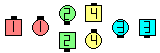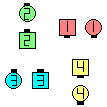(Siamese) Twosome Ah So\$B\$NA0(B \$B8e(B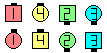\$B\$B;O\$a\$N(B formation: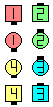Tandem TwosomeSingle Wheel\$B\$N8e(B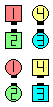Couples TwosomeSingle Wheel\$B\$N8e(B

• There are several ways to think about Twosome: the idea originated from watching a pair of ice skaters doing 'shadow' maneuvers. While the skaters move, they can make a complete 360° turn, and yet the skater that started closest to the north side of the ring is still closest to the north side of the ring, and the other skater is closest to the south side of the ring. EN: 50
Twosome \$B\$K\$D\$\$\$F(B, \$B\$\$\$/\$D\$+\$N9M\$(\$,\$"\$j\$^\$9(B. \$B%"%\$%9!&%9%1!<%?!<\$N%Z%"\$NF0\$-\$r8+\$k\$H(B, 2 \$B?M\$G(B 360° \$B2s\$k\$H\$-(B, \$BKLB&\$K6a\$\$?M\$OKLB&\$K6a\$\$\$3\$H\$rJ]\$D\$h\$&\$K2s\$j(B, \$BFnB&\$K6a\$\$?M\$OFnB&\$K6a\$\$\$3\$H\$rJ]\$D\$h\$&\$K2s\$k\$3\$H\$,\$"\$j\$^\$9(B. JP: 50
• One could also imagine a bar suspended over the Twosome dancers with strings attached to their heads like marionettes. The bar is rigid and unbreakable and, like a compass needle, always points in the same orientation. The dancers themselves can freely and individually turn suspended on their own string, but they can not change the direction in which the bar is oriented. EN: 60
Twosome \$B\$N(B 2 \$B?M\$N>e\$K2#K@\$re\$I\$&\$7\$r(B, \$BA`\$j?M7A\$N\$h\$&\$K(B, \$B;e\$G7k\$s\$G\$_\$^\$7\$g\$&(B. \$B2#K@\$O(B, \$B!V7x\$/(B -- \$B2u\$l\$J\$/(B -- \$B<'@P\$N?K\$N\$h\$&\$KF1\$8J}\$r;X\$7B3\$1\$k!W(B \$B\$H\$7\$^\$7\$g\$&(B. \$B3F!9\$N?M\$O(B, \$B<+M3\$K0l?M\$G(B, \$B<+J,\$N;e\$N2<\$G2s\$l\$^\$9\$,(B, \$B2#K@\$,;X\$7\$F\$\$\$kJ}8~\$rJQ\$(\$k\$3\$H\$O\$G\$-\$^\$;\$s(B. JP: 60
• Another way to think of Twosome is to imagine that the other dancer in your Twosome unit is like your counterpart in another square. If you start out as the #2 Lady, your counterpart in the adjacent square is also the #2 Lady. As you execute each call, imagine the other square moving closer and closer to your square, with your counterpart eventually moving adjacent to you. Even though you are both doing the exact same calls and movements, you can never switch places into the other square, so your counterpart will always be on the same side of you. EN: 70
Twosome \$B\$N\$^\$H\$^\$j\$NAjo\$K<+J,\$+\$i8+\$FF1\$8J}!W\$K\$\$\$^\$9(B. JP: 70
Dancing Hints: EN: 80
\$B%@%s%9\$N%R%s%H(B: JP: 80
• Before doing a Twosome movement, check to see which wall you are closest to, relative to the other dancer in your Twosome unit. After completion of the movement, you should still be closest to that wall. EN: 90
Twosome \$B\$NF0\$-\$r;O\$a\$kA0\$K(B, Twosome \$B\$N\$^\$H\$^\$j\$NAjJP: 90
• When dancing as a Twosome, do not firmly connect with the other dancer in your unit as you would with As Couples (holding adjacent hands) or In Tandem (placing a hand on the Leader's back). If you physically connect with the other dancer, you will be unable to dance properly since there will be a strong tendency to turn as a (solid) unit. When dancing Twosome, turning movements are always done individually! EN: 100
Twosome \$B\$rMY\$k\$H\$-\$O(B, As Couples (\$BNY\$N?M\$H\$N?M\$HJ*M}E*\$K\$D\$J\$,\$C\$F\$\$\$k\$H(B, solid \$B\$N\$^\$H\$^\$j\$G2s\$m\$&\$H\$9\$k6/\$\$798~\$,\$"\$k\$N\$G(B, \$B@5\$7\$/MY\$k\$3\$H\$,\$G\$-\$J\$/\$J\$k\$G\$7\$g\$&(B. Twosome \$B\$rMY\$k\$H\$-\$K\$O(B, \$B2s\$kF0:n\$O>o\$K(B 1 \$B?M\$G(B (individually) \$B9T\$\$\$^\$9(B. JP: 100
• When I dance Twosome, I concentrate on not letting the other dancer get past me to my closest wall. I pretend I'm trying to keep my wife, with my credit card, from entering a fancy department store. EN: 110
\$B;d\$,(B Twosome \$B\$rMY\$k\$H\$-(B, \$B\$b\$&(B 1 \$B?M\$N%@%s%5!<\$,<+J,\$K6a\$\$JI\$NJ}\$X<+J,\$r1[\$(\$F\$\$\$+\$J\$\$\$h\$&\$K(B, \$BCm0U\$r=8Cf\$5\$;\$^\$9(B. \$B;d\$N(B\$B%/%l%8%C%H%+!<%I\$r;}\$C\$F\$\$\$k:J\$r(B, \$B9b5i%G%Q!<%H\$XF~\$i\$J\$\$\$h\$&\$K\$H(B, \$B5wN%\$rCV\$\$\$F8+JP: 110

Since all calls can ultimately be broken down into a few simple movements (such as individually turn 1/4 Right|Left, Hinge, etc.), dancers should thoroughly understand how to do the following basic Twosome movements: EN: 120
\$B%3!<%k\$O(B, \$B7k6I\$N\$H\$3\$m?t>/\$J\$\$\$\$\$/\$D\$+\$N4JC1\$JF0\$-(B (\$BNc\$(\$P(B 1 \$B?M\$G1&\$^\$?\$O:8\$X(B 1/4 \$B2s\$k\$3\$H(B, Hinge \$B\$J\$I(B) \$B\$GJ,2r\$9\$k\$3\$H\$,\$G\$-\$k\$N\$G(B, \$B%@%s%5!<\$O(B, \$B0J2<\$N4pK\E*\$J(B Twosome \$B\$NF0\$-\$r\$I\$N\$h\$&\$K9T\$&\$+\$K\$D\$\$\$F(B, \$BE0DlE*\$KM}2r\$9\$Y\$-\$G\$9(B. JP: 120

• Twosome Roll, 1/4 Right, 1/4 Left, \$B\$^\$?\$O(B U-Turn Back:
Individually do the given call (since these calls are done in place). EN: 130
1 \$B?M\$GM?\$(\$i\$l\$?%3!<%k\$r\$7\$^\$9(B (\$B\$3\$l\$i\$N%3!<%k\$O\$=\$N>l\$G9T\$&\$N\$G(B). JP: 130
• Tandem Twosome Hinge:
From a Mini-Wave Box: EN: 140
Mini-Wave Box \$B\$+\$i\$O(B: JP: 140
From Tandem Couples: EN: 150
Tandem Couples \$B\$+\$i\$O(B: JP: 150
1/4 In; Veer Left.
• Couples Twosome Hinge:
From a One-Faced Line: EN: 160
One-Faced Line \$B\$+\$i\$O(B: JP: 160
1/2 Tag.
From a Two-Faced Line: EN: 170
Two-Faced Line \$B\$+\$i\$O(B: JP: 170
Any Shoulder 1/2 Tag.

{n}-some (or 1 By {n}-some) [C4]:
(where n is usually 3 or 4): n dancers, who must be relatively close to each other (having no other dancers between them) and facing toward the same direction, work as a unit following the rules for Twosome. EN: 810
(\$B\$3\$3\$G(B n \$B\$OIaDL(B 3 \$B\$^\$?\$O(B 4): n \$B?M\$,(B, \$BB>\$HHf\$Y\$F\$*8_\$\$\$K6a\$/\$K\$\$\$F(B, \$BF1\$8J}\$r8~\$\$\$F\$\$\$F(B, \$B<+J,\$?\$A\$N4V\$KC/\$b\$\$\$J\$\$\$H\$-(B, Twosome \$B\$N%k!<%k\$K=>\$C\$F\$R\$H\$^\$H\$^\$j\$GF0\$-\$^\$9(B. JP: 810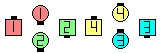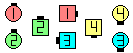1 By 3-Some Switch To A Diamond\$B\$NA0(B \$B8e(B

The above example could also be called as: EN: 180
\$B>e\$NNc\$OJP: 180
Inside Triangle work 3-some: all Switch To A Diamond.

Note: Be aware that the term 4-some may sometimes be used in circumstances not related to Twosome. For example, the caller might say 'Each 4-some' to tell the dancers on each side of the set to work together. Another example of this is when some callers use the term 'Interlocked Foursome' when they should use a more explicit term such as 'Interlocked Box'. EN: 190
\$BCm4-some \$B\$N8@MU\$O(B, \$B\$H\$-\$K\$O(B Twosome \$B\$K4X78\$7\$J\$\$>u67\$G;H\$o\$l\$k\$3\$H\$KCm0U\$7\$F\$/\$@\$5\$\$(B. \$BNc\$(\$P(B, \$B%3!<%i!<\$,(B set \$B\$N(B each side \$B\$,0l=o\$KF0\$/\$H\$\$\$&\$3\$H\$rEA\$(\$k\$?\$a\$K(B, 'Each 4-some' \$B\$H8@\$&\$3\$H\$,\$"\$j\$^\$9(B. \$BF1MM\$NJL\$NNc\$G\$O(B, 'Interlocked Box' \$B\$N\$h\$&\$K\$b\$C\$H\$O\$C\$-\$j\$H8@\$&\$Y\$-\$H\$3\$m\$r(B, \$B\$"\$k%3!<%i!<\$O(B 'Interlocked Foursome' \$B\$N8@MU\$r;H\$\$\$^\$9(B. JP: 190

Skewsome CONCEPT [C4] (Vic Ceder 1989):
Diagonal dancers work as a Twosome. EN: 809
\$BJP: 809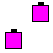or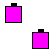Boxsome CONCEPT [C4]:
A 2 x 2 Matrix of dancers and phantoms work as a 4-some. This concept is used from a 2n x 2m Matrix (usually 2 x 8 or 4 x 4), and is typically called as 'In Your 4 x 4 Matrix: work Boxsome...' EN: 808
2 x 2 \$B\$K\$\$\$k%j%"%k\$N?M\$H(B phantom \$B\$,(B 4-some \$B\$GF0\$-\$^\$9(B. \$B\$3\$N%3%s%;%W%H\$O(B 2n x 2m Matrix (\$BIaDL(B 2 x 8 \$B\$^\$?\$O(B 4 x 4), \$B\$+\$i;H\$o\$l(B, \$B4pK\E*\$K\$O(B 'In Your 4 x 4 Matrix: work Boxsome...' \$B\$N\$h\$&\$K%3!<%k\$5\$l\$^\$9(B. JP: 808

Skewsome | Boxsome Peel & Trail: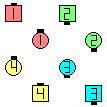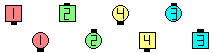Skewsome Peel & Trail\$B\$NA0(B \$B8e(B

Gruesome Twosome CONCEPT [C4]:
Usually from a 2 x 8. Same as Phantom Couples Twosome working in Parallel Waves. Also known as Couples Twosome Phantom Waves. EN: 807
\$BIaDL(B 2 x 8 \$B\$+\$i(B. Phantom Couples Twosome \$B\$G(B, \$BJ?9T\$J(B Wave \$B\$G\$N%3!<%k\$r9T\$&\$3\$H\$HF1\$8\$G\$9(B. Couples Twosome Phantom Waves \$B\$H\$7\$F\$bCN\$i\$l\$F\$\$\$^\$9(B. JP: 807Choreography for Twosome ConceptComments? Questions? Suggestions?

https://www.ceder.net/def/twosome.php?language=japan&action=edit
15-December-2019 09:52:21